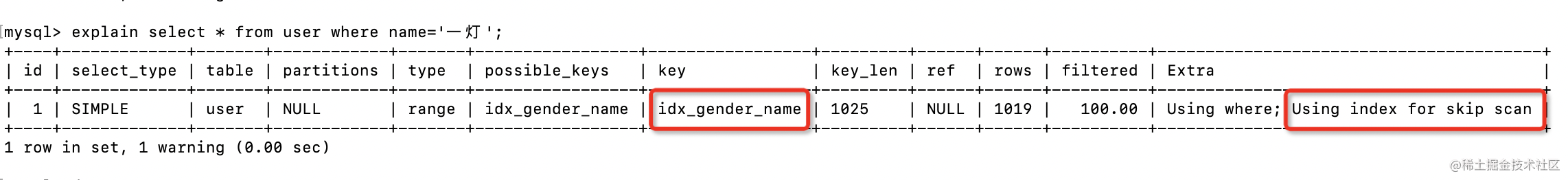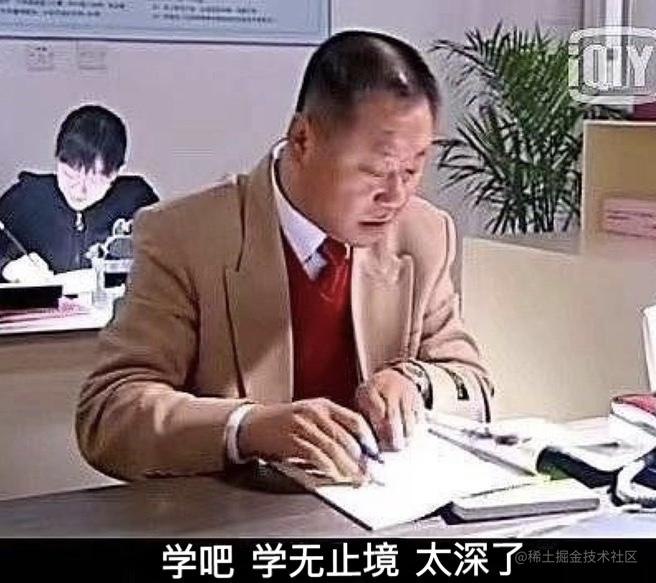# 我說MySQL聯合索引遵循最左字首匹配原則，面試官讓我回去等通知```select * from table_name where a=?; select * from table_name where a=? and b=?; select * from table_name where a=? and b=? and c=?;```

```select * from table_name where b=?; select * from table_name where c=?; select * from table_name where b=? and c=?;```MySQL8.0版本開始增加了索引跳躍掃描的功能，當第一列索引的唯一值較少時，即使where條件沒有第一列索引，查詢的時候也可以用到聯合索引。

```CREATE TABLE `user` (  `id` int NOT NULL AUTO_INCREMENT COMMENT '主鍵',  `name` varchar(255) NOT NULL COMMENT '姓名',  `gender` tinyint NOT NULL COMMENT '性別',  PRIMARY KEY (`id`),  KEY `idx_gender_name` (`gender`,`name`) ) ENGINE=InnoDB COMMENT='使用者表';```

`explain select * from user where name='一燈';``select * from user where gender in (0,1) and name='一燈';`